Looking to lay the groundwork for multiplication? Easy – use repeated addition!Author
Chal EmeryExpert Reviewer

Published: September 1, 2023Looking to lay the groundwork for multiplication? Easy – use repeated addition!Author
Chal EmeryExpert Reviewer

Published: September 1, 2023Looking to lay the groundwork for multiplication? Easy – use repeated addition!Author
Chal EmeryExpert Reviewer

Published: September 1, 2023Key takeaways

• It’s the practice of adding up equal numbers together – It sounds simple, but it’s a building block for important mathematical concepts.
• Repeated addition helps kids understand how multiplication works – This concept provides an excellent bridge between adding and multiplying.
• Repeated addition is also helpful for solving multiplication word problems – Rather than trying to do too many things at once, it gives students an easy way to break problems down into simple addition.

By now, you’ve probably learned how to add and subtract. You may have even learned about multiplication, a skill that makes you find the product of two numbers. But what is multiplication, really?

If you are struggling to understand multiplication, you can definitely use repeated addition to help you. After all, it’s taking equal groups and adding them together. But what does that mean? Let’s explore more about repeated addition.Repeated addition is the idea of adding multiple identical numbers or groups of numbers together that have the same value. This is a really helpful math skill to learn if you want to begin practicing multiplication, as both multiplication and repeated addition follow some of the same rules!

Think of repeated addition as a simple way to combine the same numbers to get a specific amount. It might seem a bit scary, but we promise learning repeated addition will be a great help as you begin to learn to multiply. Let’s take a look at a few examples of repeated addition below.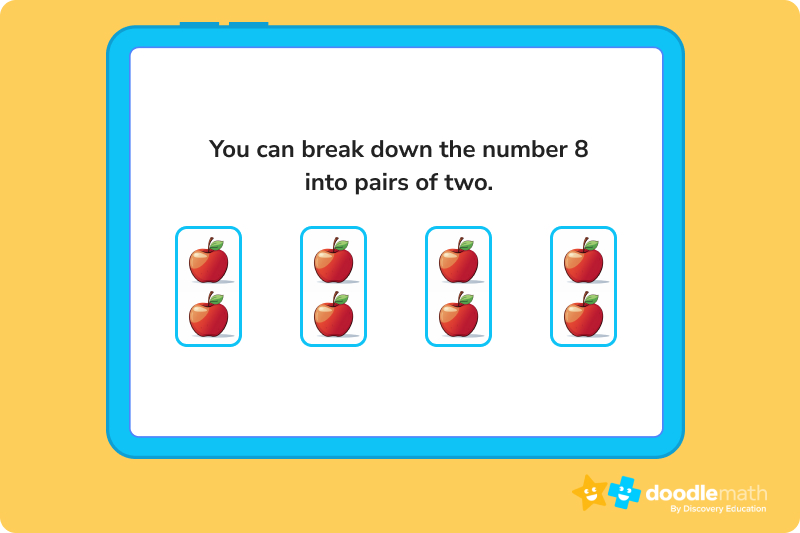### Why is repeated addition important?Repeated addition is an excellent gateway into teaching students a good foundation behind multiplication. It helps students link the ideas of addition and multiplication together by showing them how to combine numbers of equal value together.

Since repeated addition builds on skills that students already have, it’s a more natural path towards understanding the more complex idea of multiplying numbers. If your student or child is struggling somewhat with the idea of multiplication, introducing repeated addition into their learning might be a helpful solution!

Keep following below as we explain the best ways to teach your learner how to use repeated addition to solve the questions that might be stumping them.The concept of repeated addition is the same as multiplication: combining identical numbers a specific amount of times. If you haven’t memorized times tables yet, think of multiplication as repeatedly adding the same numbers together and the many sums they create.

This will make it easier to understand how multiplied values–or products–are formed. This process works for both small and large numbers; all you need is addition to figure out the right answer.

If multiplication seems a bit frightening in the beginning, use repeated addition as a helpful bridge towards understanding it better.

You’re having a slumber party and your friends want to order pizza. When the pizza comes, you have to decide how many slices to cut. You have 5 friends over. Discover how many slices to cut the pizza into by using repeated addition.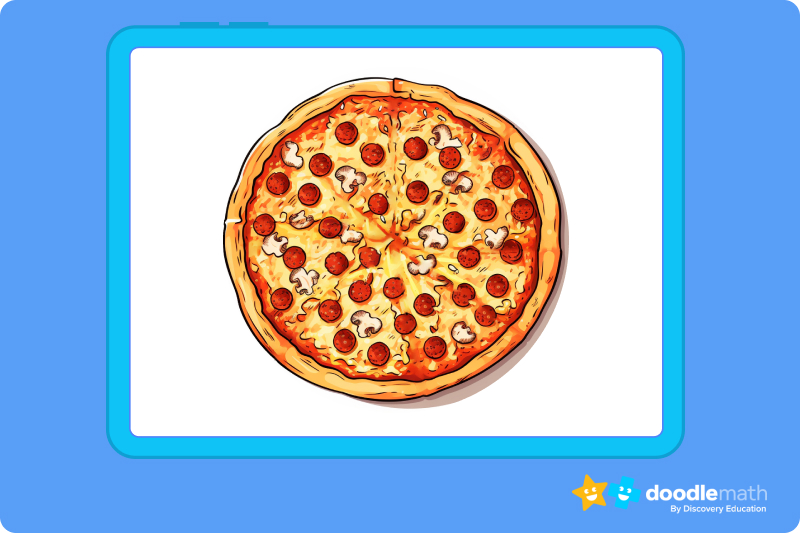Using repeated addition, we know that everyone gets 2 slices! Yum!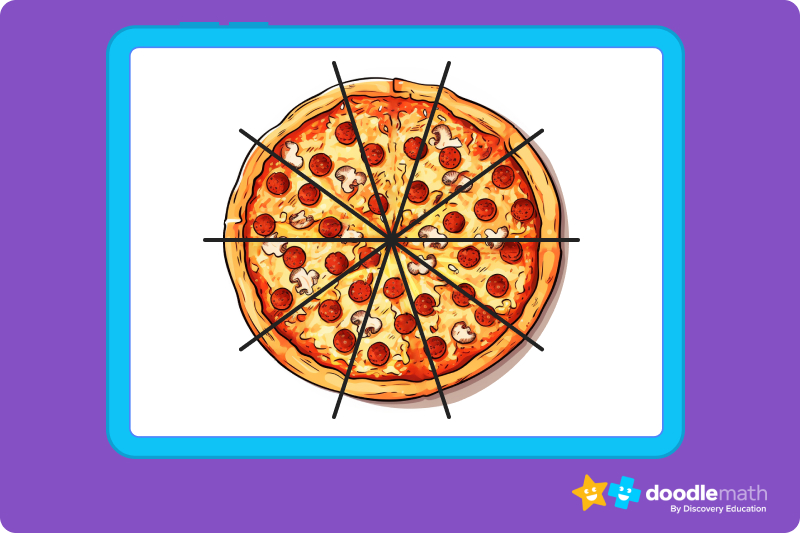Look! Up in the sky! Geese are flying in a V. You really want to know how many geese there are.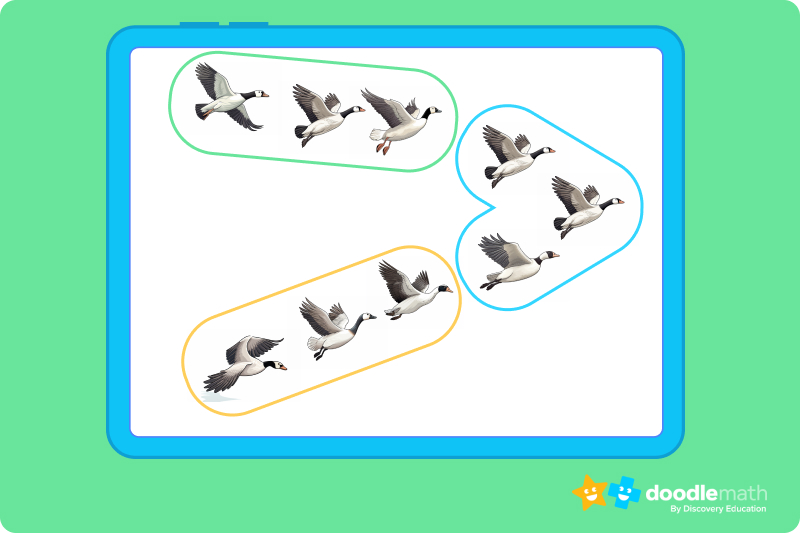There are 9 geese!

## Using repeated addition to solve word problemsWord problems are an especially difficult part of learning, because they force you to put both your reading and math skills to the test. But don’t worry, repeated addition can help you solve word problems as easily as it can help you solve numerical problems.

The same basic principles apply: find out which number or group of numbers you need to add, then find out how many times you’ll need to add that number to itself to get the correct answer.

Word problems might ask you to apply this to a range of objects, from circles to fruits to cars. But don’t let that scare you away, either. Just focus on determining which specific numbers need to be added together and how many times you’ll need to add them, and you’ll be just fine!

## Step-by-step process for solving a word problem using repeated additionHere is the word problem:

Thomas has 3 bags of apple slices. Each bag has 8 apple slices inside of it. How many apple slices does Thomas have in total?

### Step 1. Figure out what needs to be added together.

Each of Thomas’ bags has eight apple slices, which means we’ll be adding the number eight repeatedly for this question.

### Step 2. Decide how many times you’ll add this number together.

Next, identify how many bags, or groups of apple slices, Thomas has. Like the question said, Thomas has three bags.

Now, we write out our equation. We know we have three bags of apple slices, and eight apple slices in each bag. Now, let’s write down an equation that shows this. It should look like this:

8 + 8 + 8

Each eight represents the amount of apples in each bag, and the number of eights in our equation (3) is equal to the number of bags Thomas has.

### Step 4. Now let’s do our repeated addition.

8 + 8 + 8 = 24

We now know that Thomas has a total of 24 apple slices.

### Step 5. Think of how repeated addition is the same as multiplying

What we just did in this word problem follows the same rules as multiplication. We multiplied the number of apple slices (8) by the number of bags each of these apple slices were placed in (3). The equation you would use to multiply these numbers would look like this:

3 X 8 = 24

Ready to give it a go?

Now that we’ve looked at a sample problem for repeated addition, we’re ready to do a few word problems on our own to see if we can put our skills to the test. Work through the following problems on your own. Feel free to look back at the practice problem above if you get a bit confused, or if you need a refresher on how repeated addition works.

Make sure not to get discouraged! Practice is a part of learning, and we promise you’ll get the hang of this in no time. Soon, adding and multiplying will be an absolute breeze! For more practice, check out our math help app.

## Practice problems

9 + 9 + 9 + 9 + 9 + 9

## Parent Guide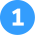How did we get here?

1. Since there are five lanes and each lane has four cars, we know we have to add four to itself five times.
2. We can write this as the following expression: 4 + 4 + 4 + 4 + 4 = 20
3. We can also express this by using a multiplication expression: 4 x 5 = 20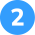The answer is 9 + 9 + 9 + 9 + 9 + 9.

How did we get here?

1. Given the multiplication expression, we know that we need to add nine to itself six times.
2. We can show that through the repeated expression written above.
3. Another correct answer would be to write six added to itself nine times, the math operates off the same logic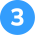The answer is 7 x 4.

How did we get here?

1. This question is asking us to relate how many berries in each box (7), there are in all boxes total (4).
2. Charles has already expressed this through repeated addition, we just need to convert it to multiplication.
3. Now, we just need to multiply the number of boxes by the number of berries in each box, giving us our answer of 4 boxes x 7 berries in each.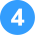4 + 4 + 4 + 4

How did we get here?

1. We know that Sally has four boxes of marbles with four marbles in each box.
2. That means we know we have to add four to itself four times.
3. We can express this through repeated addition, writing 4 + 4 + 4 + 4 to show us how many marbles Sally has across each of her boxes.Repeated addition involves adding a number together repeatedly. We use repeated addition when ew need to find the total sum of equal groups or repeated quantities. For example, 3 + 3 + 3 can be expressed as repeated addition, which is 3 groups of 3, or 3 times 3.

Repeated addition can be used to solve multiplication problems by breaking down the multiplication into adding the same number repeatedly. For instance, to solve 4 x 3, you can use repeated addition by adding 4 three times: 4 + 4 + 4 = 12. Therefore, 4 x 3 is equal to 12.

Repeated addition and division are what we call inverse operations. When using repeated addition, you add the same number repeatedly to find a total.

In division, you start with a total and split it into equal groups or parts. For example, if you have 12 objects and want to divide them equally into 3 groups, you can use repeated subtraction to find that each group will have 4 objects: 12 – 4 – 4 – 4 = 0.## Related Posts

Title 1

Title 2

Title 3

Lesson credits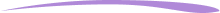Chal Emery

Chal Emery graduated from the University of North Carolina at Chapel Hill with a Bachelor’s in Global History and Political Science. Outside of writing, he enjoys long drives through spectacular country, hanging out with his friends, and spending time getting lost in a decent film or TV show.

Jill Padfield has 7 years of experience teaching high school mathematics, ranging from Alegra 1 to AP Calculas. She is currently working as a Business Analyst, working to improve services for Veterans while earning a masters degree in business administration.

Chal Emery

Chal Emery graduated from the University of North Carolina at Chapel Hill with a Bachelor’s in Global History and Political Science. Outside of writing, he enjoys long drives through spectacular country, and spending time getting lost in a decent film.

Jill Padfield has 7 years of experience teaching high school mathematics, ranging from Alegra 1 to AP Calculas. She is currently working as a Business Analyst, working to improve services for Veterans while earning a masters degree in business administration.# Are you a parent, teacher or student?

Are you a parent or teacher?

## Hi there!

Book a chat with our team